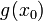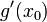# Using the product rule for differentiation for limiting behavior at points with undefined derivative$f(x_0)$$g(x_0)$$f'(x_0)$$g'(x_0)$ Conclusion about$(f \cdot g)'(x_0)$ Explanation
nonzero nonzero and same sign as$f(x_0)$ vertical tangent vertical tangent of same type as for$f$ (i.e., either both are increasing or both are decreasing) vertical tangent, type (increasing/decreasing) is determined by signs of$f,g$ and types of vertical tangent for$f,g$ [SHOW MORE]
nonzero nonzero and opposite sign to$f(x_0)$ vertical tangent vertical tangent of same type as for$f$ (i.e., either both are increasing or both are decreasing) insufficient information [SHOW MORE]
nonzero nonzero and same sign as$f(x_0)$ vertical tangent vertical tangent of opposite type as for$f$ (i.e., one is increasing and one is decreasing) insufficient information
nonzero nonzero and opposite sign to$f(x_0)$ vertical tangent vertical tangent of opposite type as for$f$ (i.e., one is increasing and one is decreasing) vertical tangent, type depends on signs